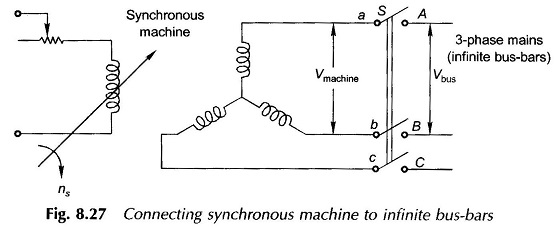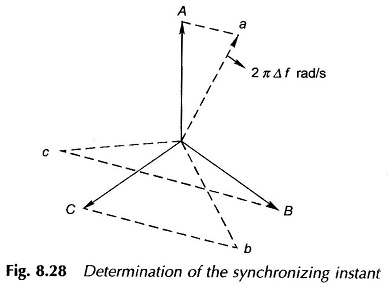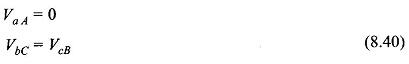## Synchronous Machine on Infinite Bus Bars:

A definite procedure has to be followed in connecting a Synchronous Machine on Infinite Bus Bars which for the present purpose will be assumed to be infinite. Infinite bus-bars means a 3-phase supply of constant voltage and frequency independent of the load exchanged (fed into the bus-bars or drawn from the bus-bars). Figure 8.27 shows a Synchronous Machine on Infinite Bus Bars with terminals a, b, c which is required to be connected to bus-bars with terminals A, B, C by means of a switch S.The machine is run as a generator with its terminals so arranged that its phase sequence is the same as that of the bus-bars. The machine speed and field current are adjusted so as to satisfy the following conditions:

• The machine terminal voltage must be nearly equal to the bus-bars voltage.
• The machine frequency is nearly equal to the bus-bars frequency, i.e. the machine speed is close to synchronous.

After the above conditions are satisfied the instant of switching on (synchronising) must be determined such that the two voltages are almost co-phasal (the acceptable phase difference is of the order of 5°). This instant is determined with the help of the method described below.

Figure 8.28 shows the phasor diagram for phase voltages (line-to-neutral) for the Synchronous Machine on Infinite Bus Bars. As the two frequencies are not exactly equal, the machine phasors are rotating slowly with respect to the bus-bar phasors at 2 π Δf rad/s, where Δf is the difference in the two frequencies. At the instant when the two sets of phasors are coincident (cophasal), the voltageThe condition can be easily determined by connecting three lamps—one across aA, the other across bC and the third across cB (in order to use standard-voltage lamps it may be necessary to employ potential transformers). The rms values of voltages V aA, VbC and VcB oscillate at the difference frequency Δf so that each lamp is alternately dark and bright. At the instant of synchronization, as per the condition (8.40) stated above, the lamp across aA is dark while the other two lamps are equally bright. It is at this instant that switch S is closed. Instead of using lamps, generating stations use an instrument called synchroscope.

Once switch S is closed the stator and rotor fields of the machine lock into each other (synchronize) and the machine then onwards runs at synchronous speed. The real power exchange with the mains will now be governed by the loading conditions on the shaft while the reactive power exchange will be determined by the field excitation.

As a generator is coupled to a prime mover it is easy to follow the above procedure to connect it to the bus-bars. The same procedure has to be followed for a synchronous motor which must be run initially by an auxiliary device (may be a small dc/induction motor) and then synchronized to the bus-bars. It may be pointed out here that the synchronous motor is non-self-starting. If, for example, switch S is closed in Fig. 8.27 with the rotor stationary, the stator and rotor fields will be moving relative to each other at synchronous speed so as to develop alternating torque with zero average value and as a result the motor would not start. Synchronous motors are made self-starting by providing short-circuited bars on the rotor which produce induction torque for starting.

Scroll to Top